# Solving Systems of Equations using Substitution

##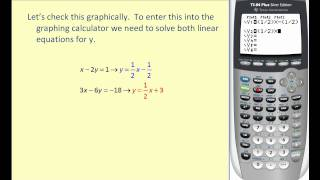By Mathispower4u

Solving Systems of Equations using Substitution# Solving Systems of Equations using Substitution

##By Mathispower4u

Solving Systems of Equations using Substitution# Solving systems of equations in three variables

##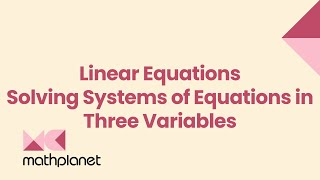By MathPlanetVideos

Solve the systems of equation in our example.# Solving systems of equations in two variables

##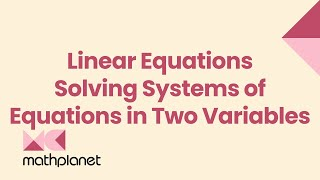By MathPlanetVideos

Solve the system of equations: {2x−4y=0 −4x+4y=−4# Ex 2: Solve a System of Equations Using Substitution

##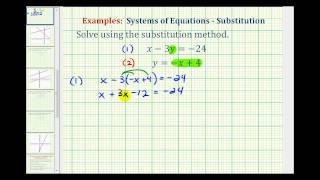By Mathispower4u

Ex 2: Solve a System of Equations Using Substitution# Solve systems of equations graphically

##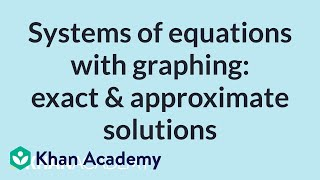By Khan Academy

Sal solves a system of two linear equations in standard form, and then approximates the solution of a system whose solution isn't clearly visible.# Example Problem - Solving a System with Linear and Quadratic Equations

##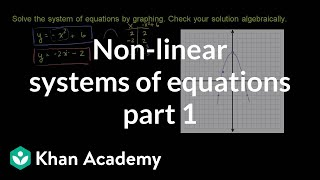By Khan Academy

Watch this example of solving a system of a linear equation and quadratic equation. The solution is found by finding the intersection of the the line and parabola graphically. Then, the solution is verified algebraically.# Ex 2: Solve a System of Equations Using Substitution

##By Mathispower4u

Ex 2: Solve a System of Equations Using Substitution# Solve systems of equations graphically

##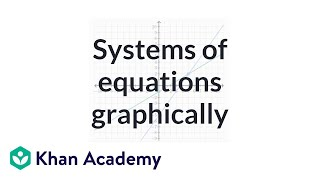By Khan Academy

Sal graphs the following system of equations and solves it by looking for the intersection point: y=7/5x-5 and y=3/5x-1.# Solve any system of two linear equations

##By Khan Academy

Sal solves a system of two linear equations in standard form, and then approximates the solution of a system whose solution isn't clearly visible.# Solve any system of two linear equations

##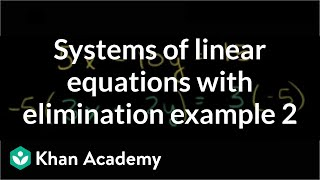By Khan Academy

Sal solves the following system of equations by manipulating it and then eliminating y: 5x-10y=15 and 3x-2y=3.# Solve any system of two linear equations

##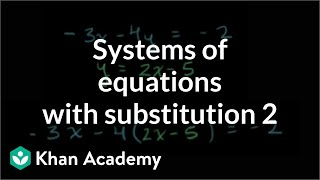By Khan Academy

Sal solves the following system of linear equations using substitution: -3x-4y=-2 and y=2x-5.# Solve systems of linear equations with elimination (basic)

##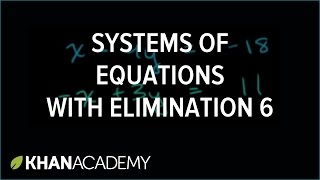By Khan Academy

Sal solves the following system of equations by eliminating x: x-4y=-18 and -x+3y=11.# Solving Linear Systems of Equations Using Substitution

##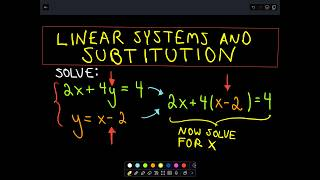By PatrickJMT

Youtube Presents Solving Linear Systems of Equations Using Substitution an educational video resources on english language arts.# Solving Linear Systems of Equations Using Substitution

##By PatrickJMT

Youtube Presents Solving Linear Systems of Equations Using Substitution an educational video resources on english language arts.# Solving Linear Systems of Equations Using Substitution

##By PatrickJMT

Youtube Presents Solving Linear Systems of Equations Using Substitution an educational video resources on english language arts.# Ex 2: Solve a System of Equations Using the Elimination Method

##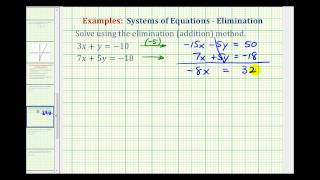By Mathispower4u

Ex 2: Solve a System of Equations Using the Elimination Method# Solve systems of linear equations with substitution

##By Khan Academy

Sal solves the following system of linear equations using substitution: -3x-4y=-2 and y=2x-5.# Solve System of Linear Equations Using Addition Method

##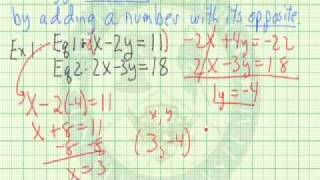By APUS07

YouTube presents Solve System of Linear Equations Using Addition Method, an educational video resource on math.# Solve any system of two linear equations

##By Khan Academy

Sal solves the following system of equations by manipulating it and then eliminating y: 5x-10y=15 and 3x-2y=3.